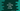# How to sort an array in ascending order in C++## How to sort an array in ascending order in C++:

We will learn how to sort an array in ascending order in C++. The program will take the total number of elements and the array elements as inputs from the user and sort the array values in ascending order. We can write our own algorithm or we can use a predefined method of STL. I will show you both.

### Example 1: C++ program to sort an array in ascending order using loops:

Let’s use two loops to sort the array in ascending order. This algorithm will compare each number of the array with all numbers to its right and move the smallest number to the start of the array on each iteration.

Below is the complete program:

``````#include <iostream>
using namespace std;

int main()
{
int t, s;

cout << "Enter the size of the array" << endl;
cin >> s;
int arr[s];

cout << "Enter the array elements: " << endl;
for (int i = 0; i < s; i++)
{
cin >> arr[i];
}

for (int i = 0; i < s; i++)
{
for (int j = i + 1; j < s; j++)
{
if (arr[i] > arr[j])
{
t = arr[i];
arr[i] = arr[j];
arr[j] = t;
}
}
}

for (int const &e : arr)
{
std::cout << e << ' ';
}
}``````

Here,

• The size of the array is stored in the variable s. We created an array arr of size s to hold the user input numbers.
• The user input elements are read and stored in the array arr.
• To sort the array, we are using two for loops.

• The outer loop runs from the start to the end element of the array.
• For each element points by the outer loop, the inner loop iterates over the other elements to the right of it. The inner loop finds the smallest element and place it to the position points by the outer loop. So, on each iteration of the outer loop, the inner loop finds the smallest element starting from the outer loop pointer to the end.
• Once the sorting is done, it uses another for loop to print the content of the array.

If you run this program, it will print output as like below:

``````Enter the size of the array
6
Enter the array elements:
10
9
8
7
6
5
5 6 7 8 9 10

Enter the size of the array
5
Enter the array elements:
1
2
10
3
2
1 2 2 3 10``````

### Example 2: By using STL sort:

The sort method of C++ STL is an inbuilt method defined to sort the content of an array. This method is defined as like below:

``sort(start, end, comp)``
• start is the start iterator of the array.
• end is the end iterator of the array.
• comp is a function that defines how the sorting should be done. It is the compare function and this is an optional value.

Let me show you how it works:

``````#include <iostream>
#include <algorithm>
using namespace std;

int main()
{
int s;

cout << "Enter the size of the array" << endl;
cin >> s;
int arr[s];

cout << "Enter the array elements: " << endl;
for (int i = 0; i < s; i++)
{
cin >> arr[i];
}

sort(arr, arr + s);

for (int const &e : arr)
{
std::cout << e << ' ';
}
}``````

We passed the pointers to the first and the last element of the array. If you run this program, it will print the sorted array.

``````Enter the size of the array
5
Enter the array elements:
5
4
3
2
1
1 2 3 4 5``````

This includes all elements in [start, end) which includes all the elements between start and end including the element pointed by start but not the element pointed by end.

We can also pass the compare function to use different ways to sort the array. This should be a function that takes two variables as its parameters and returns one boolean value. If the return value is true, the value passed to the first parameter will go before the second parameter value. Else, the second one will go before the first.

For example:

``````#include <iostream>
#include <algorithm>
using namespace std;

bool compare(int i, int j) { return i > j; }
int main()
{
int s;

cout << "Enter the size of the array" << endl;
cin >> s;
int arr[s];

cout << "Enter the array elements: " << endl;
for (int i = 0; i < s; i++)
{
cin >> arr[i];
}

sort(arr, arr + s, compare);

for (int const &e : arr)
{
std::cout << e << ' ';
}
}``````

This program will sort the array elements in descending order.